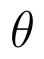Select Page

# 12 Science Maths CBSE Answers for MCQ Differential Equations in English

12 Science Maths CBSE Answers for MCQ Differential Equations in English to enable students to get Answers in a narrative video format for the specific question.

Expert Teacher provides 12 Science Maths CBSE Answers for MCQ Differential Equations through Video Answers in English language. This video solution will be useful for students to understand how to write an answer in exam in order to score more marks. This teacher uses a narrative style for a question from Differential Equations not only to explain the proper method of answering question, but deriving right answer too.

Please find the question below and view the Answer in a narrative video format.

Question:

Answer Video in English:

You can select video Answers from other languages also. Please check Answers in ( Hindi )

## Similar Questions from CBSE, 12th Science, Maths, Differential Equations

Question 1 : If x cos(a + y) = cos y, then prove thatHence show that(View Answer Video)

Question 2 : Find the particular solution of the differential equationgiven that y = 1 when x = 0. (View Answer Video)

Question 3 : Find the general solution of differential equation(View Answer Video)

Question 4 : Find the particular solution of the differential equationgiven that y = 1, when x = 0. (View Answer Video)

Question 5 : If m and n are the order and degree, respectively of the differential equationthen write value of m+n. (View Answer Video)

### Continuity and Differentiability

Question 1 : Differentiate the functionwith respect to x. (View Answer Video)

Question 2 : Find the value of k, if the area of the triangle is 4 sq unit and vertices are (-2, 0), (0, 4), (0, k). (View Answer Video)

Question 3 :  Differentiate w.r.t.x the function. (View Answer Video)

Question 4 : Findfor the function. (View Answer Video)

Question 5 : Differentiate the function w.r.t.x. (View Answer Video)

### Vector Algebra

Question 1 : Find the vector quantities from the following:
(i) Time period          (ii) Distance          (iii) Force
(iv) Velocity               (v) Work done

Question 2 : Find the projection of the vectoron the vector(View Answer Video)

Question 3 : Write the position vector of the point which divides the join of points with position vectorsandin the ratio 2:1.  (View Answer Video)

Question 4 : If a line has direction ratios 2, -1, -2, then what are its direction cosines?   (View Answer Video)

Question 5 : Find a unit vector parallel to the sum of vectorsand(View Answer Video)

### Three Dimensional Geometry

Question 1 : Write the vector equation of the plane passing through the point (a, b, c) and parallel to the plane(View Answer Video)

Question 2 : Write the sum of intercepts cut off by the planeon the three axes.  (View Answer Video)

Question 3 : If a line marks anglesandwith x, y and z-axis respectively, whereis acute, then find. (View Answer Video)

Question 4 : If linesandintersect, then find the value of k and hence find the equation of plane containing these lines. (View Answer Video)

Question 5 : Find the equation of the plane passing through the line of intersection of the planes x + y + z = 1 and 2x + 3y + 4z = 5 which is perpendicular to the plane x - y + z = 0. Also, find the distance of the plane obtained above from the origin. (View Answer Video)